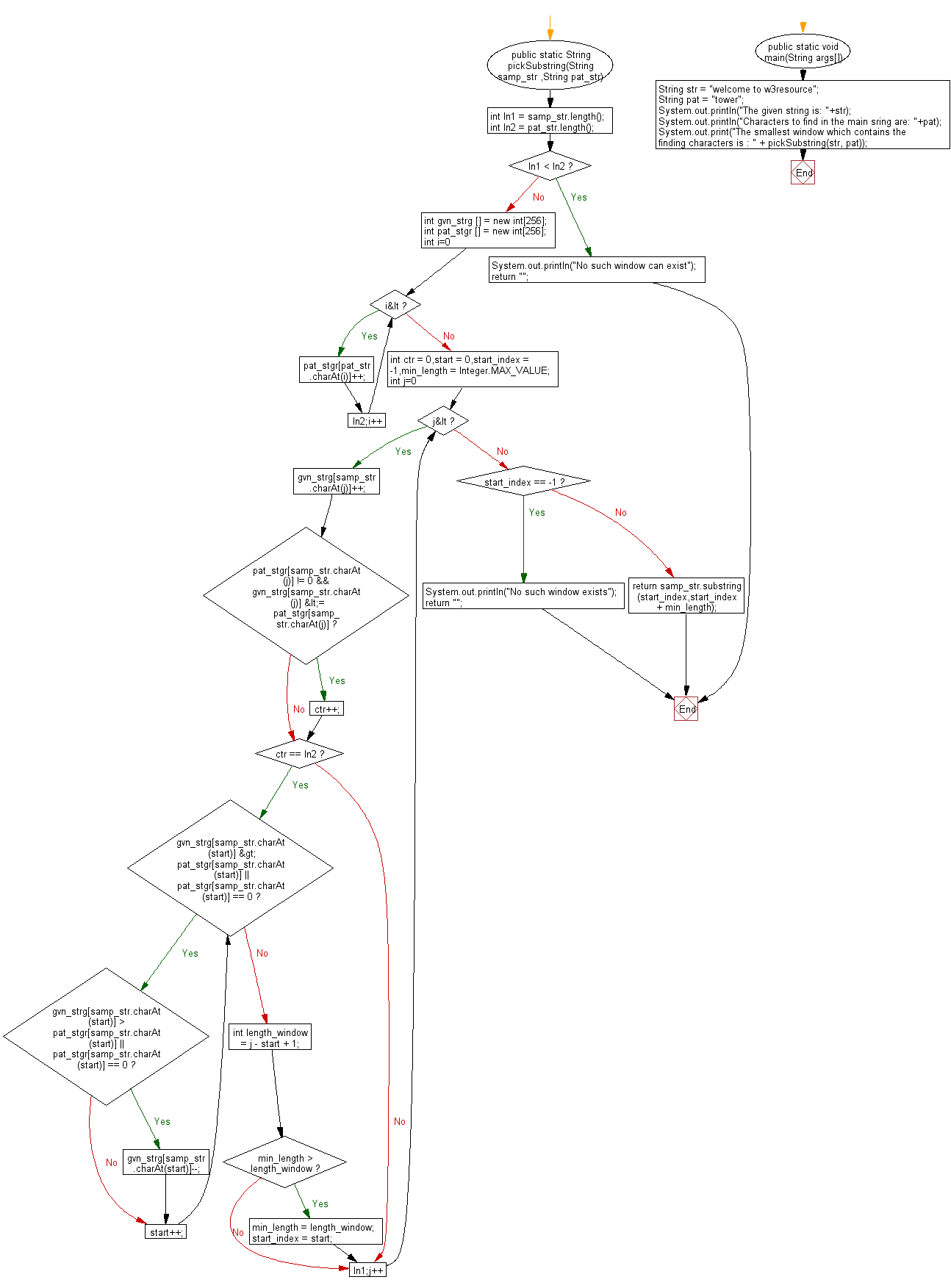﻿ Java exercises: Find the smallest window in a string containing all characters of another string - w3resource# Java String Exercises: Find the smallest window in a string containing all characters of another string

## Java String: Exercise-54 with Solution

Write a Java program to find the smallest window in a string containing all characters of another string.

Sample Solution:

Java Code:

``````import java.util.*;
class Main
{
public static String pickSubstring(String samp_str ,String pat_str)
{
int ln1  = samp_str.length();
int ln2  = pat_str.length();
if(ln1 < ln2)
{
System.out.println("No such window can exist");
return "";
}
int gvn_strg [] = new int;
int pat_stgr [] = new int;
for(int i=0;i<ln2;i++)
pat_stgr[pat_str.charAt(i)]++;
int ctr = 0,start = 0,start_index = -1,min_length = Integer.MAX_VALUE;
for(int j=0;j<ln1;j++)
{
gvn_strg[samp_str.charAt(j)]++;
if(pat_stgr[samp_str.charAt(j)] != 0 && gvn_strg[samp_str.charAt(j)] <= pat_stgr[samp_str.charAt(j)])
ctr++;
if(ctr == ln2)
{
while(gvn_strg[samp_str.charAt(start)] > pat_stgr[samp_str.charAt(start)] || pat_stgr[samp_str.charAt(start)] == 0)
{
if(gvn_strg[samp_str.charAt(start)] > pat_stgr[samp_str.charAt(start)] || pat_stgr[samp_str.charAt(start)] == 0)
gvn_strg[samp_str.charAt(start)]--;
start++;
}
int length_window = j - start + 1;
if(min_length > length_window)
{
min_length = length_window;
start_index = start;
}
}
}
if(start_index == -1)
{
System.out.println("No such window exists");
return "";
}
return samp_str.substring(start_index,start_index + min_length);
}
public static void main(String args[])
{
String str = "welcome to w3resource";
String pat = "tower";
System.out.println("The given string is: "+str);
System.out.println("Characters to find in the main string are: "+pat);

System.out.print("The smallest window which contains the finding characters is : " + pickSubstring(str, pat));
}
}
```
```

Sample Output:

```The given string is: welcome to w3resource
Characters to find in the main string are: tower
The smallest window which contains the finding characters is : to w3re
```

Flowchart:Java Code Editor:

Improve this sample solution and post your code through Disqus

What is the difficulty level of this exercise?

﻿

New Content: Composer: Dependency manager for PHP, R Programming